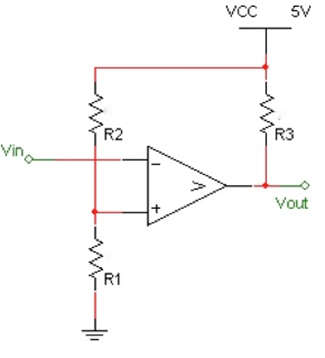Create an Account

Home / Questions / For the comparator below complete the design so that the threshold voltage is 1 25V which ...

For the comparator below complete the design so that the threshold voltage is 1 25V which means that if the input is below

For the comparator below, complete the design so that the threshold voltage is 1.25V which means that if the input is below 1.25V, the output will be HIGH and if its greater, it will be low. Once the resistance values are found, use Multisim to validate the design by applying a sine wave of 3Vpp into Vin and plot the results.Using the 8 bit Digital-to-Analog (VDAC) in Multisim, design the part with a 12V reference. Next apply the following inputs in the table below and both calculate and measure the output voltage. What is the resolution of the DAC? Show your circuit and results in Multisim.

 Digital Inputs Calculated Analog Output Voltage Measured Analog Output Voltage 7 6 5 4 3 2 1 0 0 0 0 0 0 0 0 1 0 0 0 0 1 0 0 1 0 0 0 1 0 0 0 0 0 0 0 1 1 1 1 1 0 1 0 1 0 1 0 1 1 0 0 0 0 0 0 0 1 1 1 1 1 1 1 1

Show transcribed image text For the comparator below, complete the design so that the threshold voltage is 1.25V which means that if the input is below 1.25V, the output will be HIGH and if its greater, it will be low. Once the resistance values are found, use Multisim to validate the design by applying a sine wave of 3Vpp into Vin and plot the results. Using the 8 bit Digital-to-Analog (VDAC) in Multisim, design the part with a 12V reference. Next apply the following inputs in the table below and both calculate and measure the output voltage. What is the resolution of the DAC? Show your circuit and results in Multisim.

Jul 31 2020 View more View LessSubscribe To Get Solution